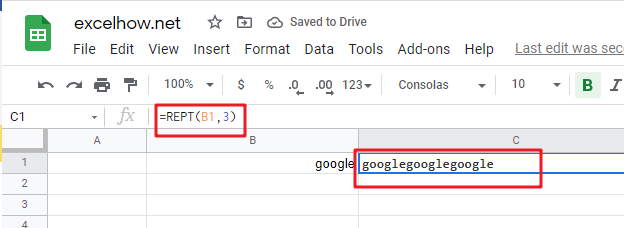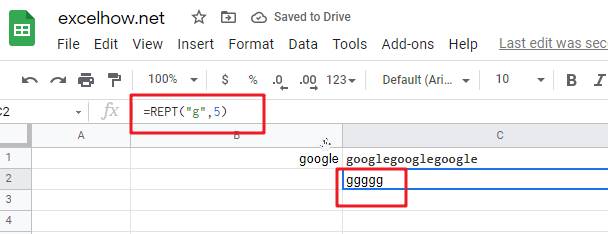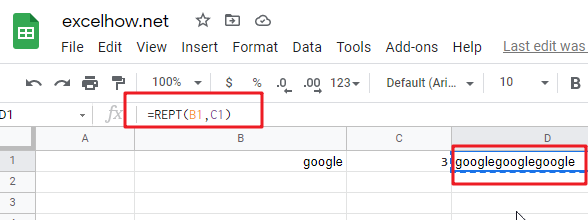This post will guide you how to use Google Sheets REPT function with syntax and examples.

## Description

The Google Sheets REPT function repeats a text string a specified number of times.

The REPT function can be used to repeat specified text repeated a number of times in google sheets. And it’s return value as the repeated text. The purpose of this function is repeat text as specified.

The REPT function is a build-in function in Google Sheets and it is categorized as a Text Function.

## Syntax

The syntax of the REPT function is as below:

= REPT (text, number_times)

Where the REPT function arguments are:

• Text -This is a required argument. The text string that you want to repeat.
• Number_times – This is a required argument.  The number of times that you want to repeat

Note:

• If number_times is zero, the REPT function will return empty text. And If `number_times` value is not an integer, it is truncated to an integer value.
• `Nuber_times` argument should be zero or a positive integer, otherwise, it will return an #VALUE error.
• REPT function takes two arguments, `text` and `number_times`. The first argument, `Text` is the characters to repeat. And the second argument, `number_times` is the number of times text should appear in the value returned.
• REPT function does not insert any space characters between repetitions of text. If you wish to insert a space, a space character must be appended to the end of the value of text value. And the resulting return value from REPT function will have at least one trailing space, and you can use another function called TRIM function to remove it.

## Google Sheets REPT Function Examples

The below examples will show you how to use google sheets REPT function to repeat a text string a given number of times.

#1 To repeat a text string in B1 cell 3 times, just using the following formula:

`=REPT (B1,3) //it returns “googlegooglegoogle”`#2 Repeat “g” letter 5 times, just using the following formula:

`=REPT(“g”,5) //it returns “ggggg”`#3 Repeat text string in Cell B1 using a specified number of times in Cell C1, you can type the following REPT formula in Cell D1:

`=REPT(B1,C1) //it returns “googlegooglegoogle”`